# Tensile and Compressive Stress and Strain Equations

Lesson Transcript
Instructor: Damien Howard

Damien has a master's degree in physics and has taught physics lab to college students.

Tensile and compressive stress and strain equations are used to find out how stiff a material is. Learn about stress and strain, tension and compression, elastic modulus and Hooke's law, and the stress-strain curve. Updated: 10/28/2021

## Stress and Strain

When looking at a force problem, you're probably used to being concerned only about how the object moves after being affected by the forces acting on it. What you probably haven't thought too much about is how the structure of the object might be affected by that same force. A common example of this happens with bridges. When people or vehicles move over a bridge, their weight creates a downwards force. The bridge doesn't change position due to this weight, but it can bend.

To help ensure that a bridge can hold the amount of weight that it was designed to withstand without breaking under heavy traffic, it undergoes something called a stress test. Stress is defined as internal force per unit area. Mathematically, we can write this as: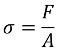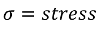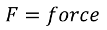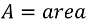The equation for stress gives it standard units of Pascals (Pa). The Pascal is a common unit used for pressure. Both pressure and stress are forces over an area. The difference between the two is that pressure is external, whereas stress is internal to the object.

The amount of stress applied to an object will determine the level of strain the object experiences, where strain is the amount of deformation an object undergoes due to stress. In essence, stress is the internal force, and strain is the physical effect of that force on the object.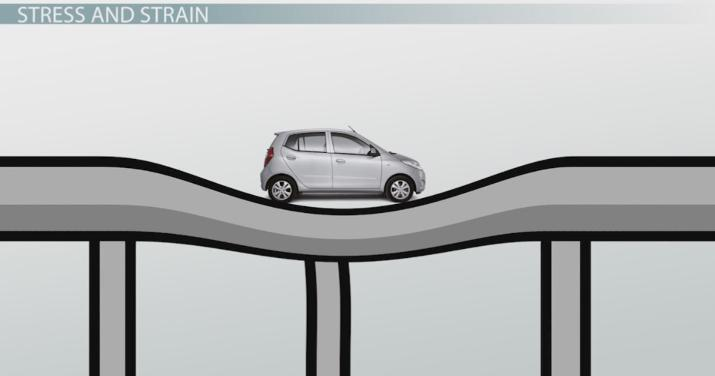An error occurred trying to load this video.

Try refreshing the page, or contact customer support.

Coming up next: Shear and Bulk Stress and Strain Equations

### You're on a roll. Keep up the good work!

Replay
Your next lesson will play in 10 seconds
• 0:00 Stress and Strain
• 1:19 Tension and Compression
• 2:24 Elastic Modulus and…
• 4:15 Stress-Strain Curve
• 5:42 Lesson Summary
Save Save

Want to watch this again later?

Timeline
Autoplay
Autoplay
Speed Speed

## Tension and Compression

Depending on how force is applied to the object, it can undergo different types of stress and strain. Two of the most common types are tensile and compressive stress and strain. When an object is under tension it is experiencing an increase in length. A rubber band being stretched out is a common example of an object experiencing tensile stress and strain. The opposite of tension is compression, where an object is undergoing a decrease in length. If you've ever squeezed a rubber ball or a pet's squeak toy in your hands, you were creating a compressive stress and strain in the object.

In the case of tensile and compressive strain, the mathematical definition is the change in length divided by the original length of the object. The difference between the two is how that length changes. For tensile it's an increase in length, and for compressive it's a decrease in length. If we want to separate them into two different equations, we can write them out as follows.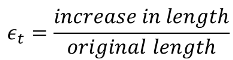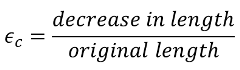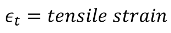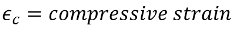## Elastic Modulus and Hooke's Law

Any object is capable of experiencing tensile and compressive stress and strain, but not all react to that stress to the same degree. Let's imagine you have two small blocks in your hands. One is made of steel and the other is rubber. If the same amount of stress is applied to both of them, which will experience more strain? Which one will stretch or compress further? You know it's going to be the rubber block. The steel block is much stiffer than the rubber block, and won't stretch or compress as much.

In physics, we can use something called the elastic modulus to measure the stiffness of a material. It turns out all we need to find the elastic modulus is the stress applied to the object and the strain it experiences. We can write elastic modulus out as the ratio of the two. So, elastic modulus equals stress divided by strain.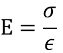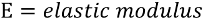The elastic modulus is actually a constant. This means any given material will always have the same elastic modulus. If we rearrange the equation to get stress by itself, we can see something interesting. We can get the equation in the form of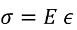It's not immediately obvious, but you may have seen an equation like this before. You have stress, a force per unit area, equal to a constant multiplied by strain, a displacement. This is very similar to Hooke's law, which you might have looked at for springs, where force equals the spring constant times a displacement.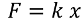It turns out that our rearranged elastic modulus equation is also Hooke's law. Our rearranged elastic modulus equation is a more general form of Hooke's law that applies to all materials, and not just springs. Hooke's law shows us that the stress needed to stretch or compress a material is directly proportional to the distance it is stretched or compressed.

## Stress-Strain Curve

Another interesting way to compare stress and strain is to look at a graph of the two.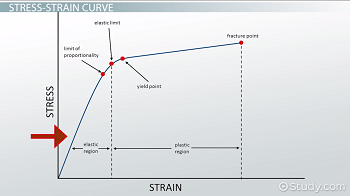To unlock this lesson you must be a Study.com Member.

### Register to view this lesson

Are you a student or a teacher?